Question

# 1b. You wish to make a 0.395 M perchloric acid solution from a stock solution of...

1b. You wish to make a 0.395 M perchloric acid solution from a stock solution of 3.00 M perchloric acid. How much concentrated acid must you add to obtain a total volume of 75.0 mL of the dilute solution?

1c. In the laboratory you dilute 4.36 mL of a concentrated 12.0 M perchloric acid solution to a total volume of 50.0 mL. What is the concentration of the dilute solution?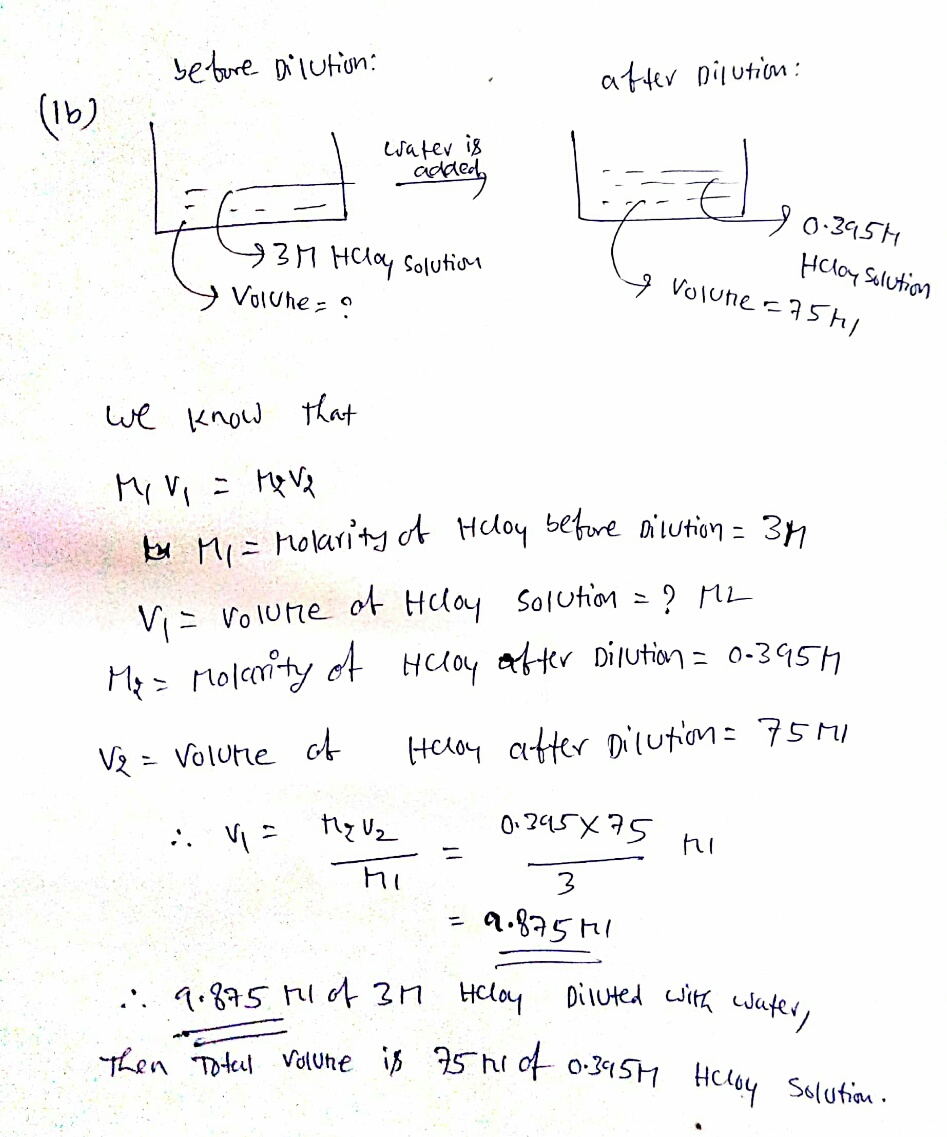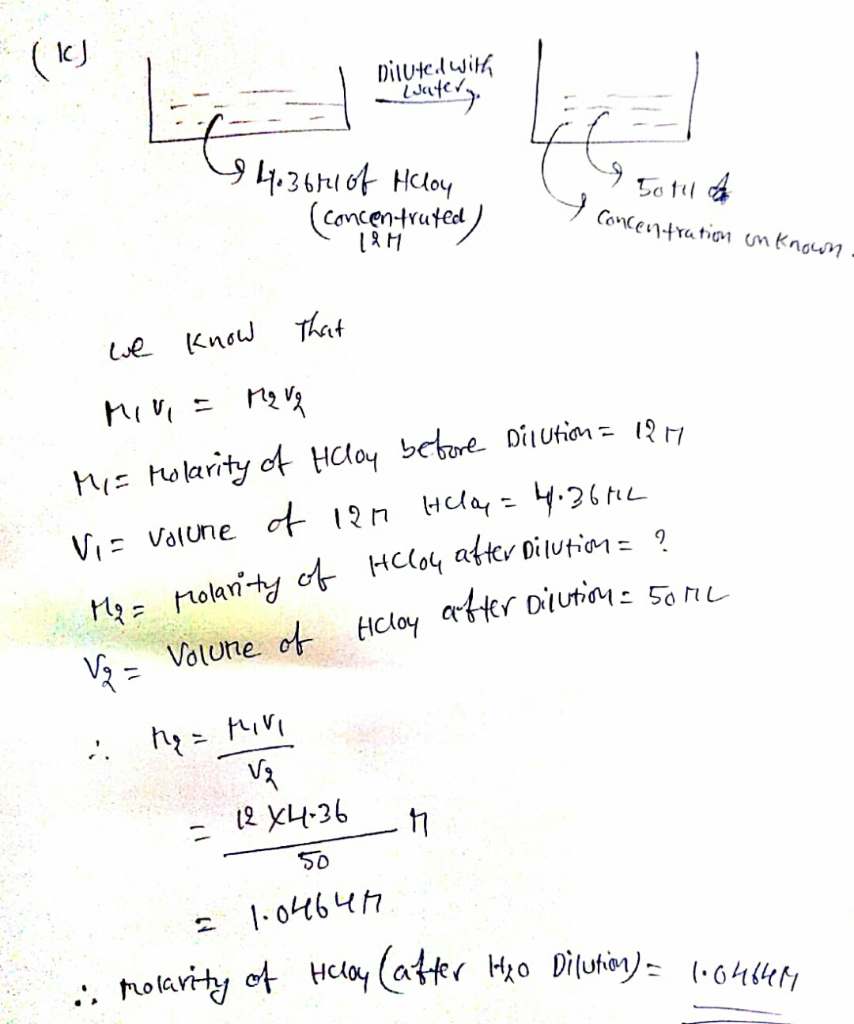#### Earn Coins

Coins can be redeemed for fabulous gifts.

Similar Homework Help Questions
• ### You wish to make a 0.249 M hydrobromic acid solution from a stock solution of 12.0...

You wish to make a 0.249 M hydrobromic acid solution from a stock solution of 12.0 M hydrobromic acid. How much concentrated acid must you add to obtain a total volume of 50.0 mL of the dilute solution? __mL You wish to make a 0.112 M nitric acid solution from a stock solution of 12.0 M nitric acid. How much concentrated acid must you add to obtain a total volume of 150 mL of the dilute solution? __mL In the...

• ### You wish to make a 0.257 M nitric acid solution from a stock solution of 3.00...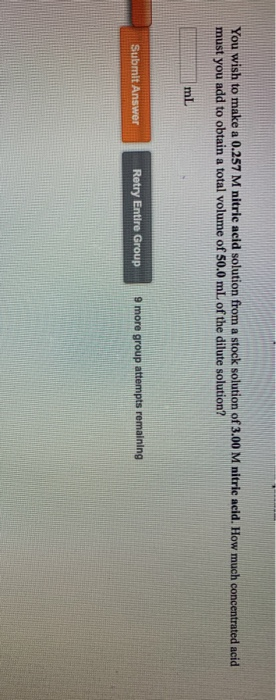You wish to make a 0.257 M nitric acid solution from a stock solution of 3.00 M nitric acid. How much concentrated acid must you add to obtain a total volume of 50.0 mL of the dilute solution? Submit Answer Retry Entire Group 9 more group attempts remaining

• ### You wish to make a 0.445 M hydrochloric acid solution from a stock solution of 12.0...

You wish to make a 0.445 M hydrochloric acid solution from a stock solution of 12.0 M hydrochloric acid. How much concentrated acid must you add to obtain a total volume of 150 mL of the dilute solution?

• ### You wish to make a 0.252 M nitric acid solution from a stock solution of 12.0...

You wish to make a 0.252 M nitric acid solution from a stock solution of 12.0 M nitric acid. How much-concentrated acid must you add to obtain a total volume of 175 mL of the dilute solution?

• ### You wish to make a 0.135 M nitric acid solution from a stock solution of 3.00...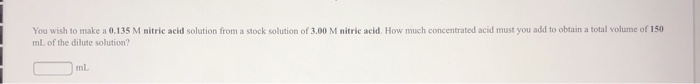You wish to make a 0.135 M nitric acid solution from a stock solution of 3.00 M nitric acid. How much concentrated acid must you add to obtain a total volume of 150 mL of the dilute solution? ml

• ### Gonca the References to importatif eded for the You wish to make a 0.266 M perchloric...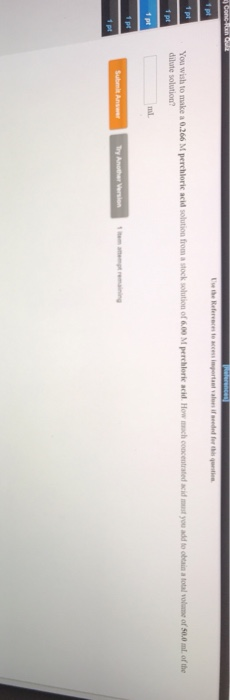Gonca the References to importatif eded for the You wish to make a 0.266 M perchloric acid solution from a stock solution of 6.00 M perchloric acid How much concentrated dilute solution you to obtain a lotal volume of 50.0 ml of the Try Another Version

• ### I do not need to see work shown, just the correct exact answers. Please be clear...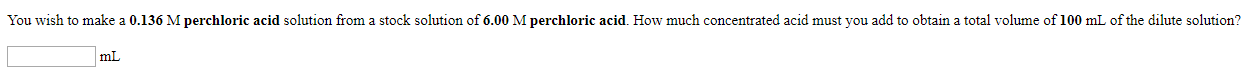I do not need to see work shown, just the correct exact answers. Please be clear on what the answers are. I will give thumbs up if correct. 4-10 You wish to make a 0.136 M perchloric acid solution from a stock solution of 6.00 M perchloric acid. How much concentrated acid must you add to obtain a total volume of 100 mL of the dilute solution? mt In the laboratory you dilute 4.47 mL of a concentrated 3.00 M...

• ### You wish to make a 0.132 M nitric acid solution from a stock solution of 6.00...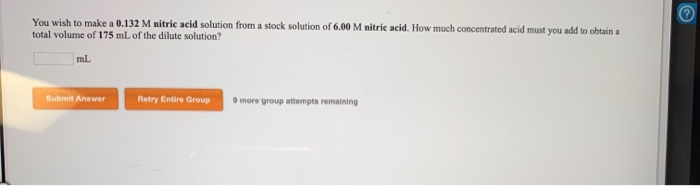You wish to make a 0.132 M nitric acid solution from a stock solution of 6.00 M nitric acid. How much concentrated acid must you add to obtain total volume of 175 mL of the dilute solution? Submit Answer Retry Entire Group more group attempts remaining 1 pts are In the laboratory a student combines 37.6 ml of a 0.289 M potassium sulfide solution with 22.5 ml of a 0.629 M potassium carbonate solution What is the final concentration of...

• ### 1A)The compound magnesium bromide is a strong electrolyte. Write the reaction when solid magnesium bromide is...

1A)The compound magnesium bromide is a strong electrolyte. Write the reaction when solid magnesium bromide is put into water: 1B)The compound zinc sulfate is a strong electrolyte. Write the reaction when solid zinc sulfate is put into water 1C)The compound cobalt(II) chloride is a strong electrolyte. Write the reaction when solid cobalt(II) chlorideis put into water: 1D)You need to make an aqueous solution of 0.171 M potassium iodide for an experiment in lab, using a 300 mL volumetric flask. How...

• ### In the laboratory, you dilute 3.61 mL of a concentrated 12.0 M hydrochloric acid solution to...

In the laboratory, you dilute 3.61 mL of a concentrated 12.0 M hydrochloric acid solution to a total volume of 175 mL. What is the concentration of the dilute solution?